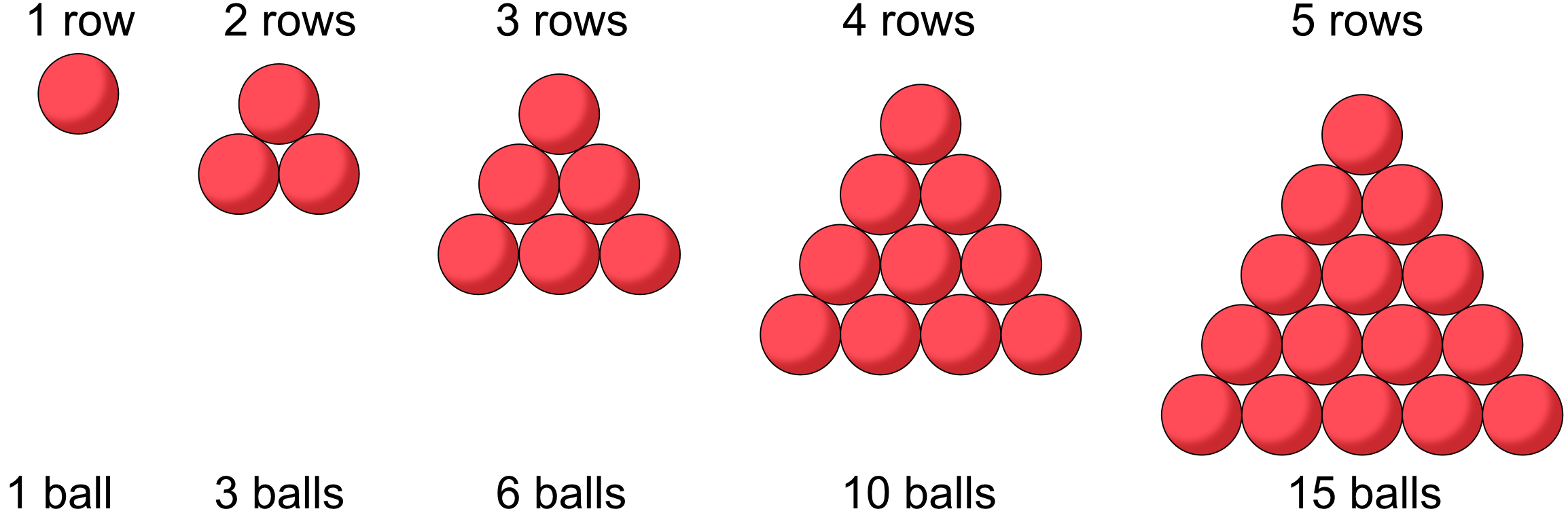Triangular Sequences

# Triangular Sequences

Basic, GCSE(F),

A triangular sequence is named after the shape that can be made by individual balls which are placed together as close as possible.

For each new row, add one additional ball to the number of balls in the previous row.Any selected row equates to the term number. The value of the term is the total number of balls in the triangle up to and inlcuding that row.

## Examples

1. What is the value of the 6th triangular number?